\$B:#>,\$N0lGU(B

\$BKhF|!":#>,\$N0lGU\$r>e\$2\$F\$\$\$^\$9!#(B
\$B:#>,0{\$`\$b\$N\$K:\$\$C\$?\$i!";29M\$K\$7\$F\$_\$F\$/\$@\$5\$\$!#(B

2022\$BG/(B7\$B7n(B

 1(IB<^D^3\$B!!(IJ_0D(B12 2(I=6X0\8^(B10\$BG/(B 3 \$B5Y\$_(B 4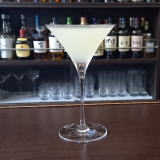(I13^(42<.](B 5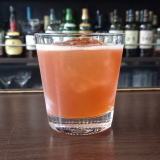(IA,0X0%A,/L_X](B 6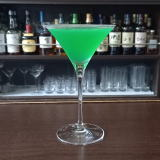(I<0%5L^%WL^(B 7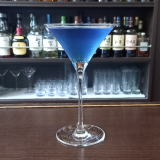(IJ_0L_Y%Z2](B 8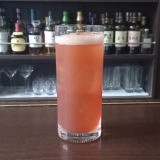(I=[0<^]%L(=^(B 9 \$B5Y\$_(B 10(I:Y:J^0D^(B 11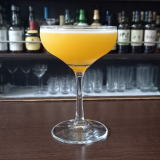(IJ\21]%68CY(B 12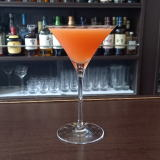(I@^L^Y%Z2]N^0(B 13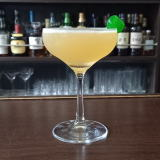(I4]D^Z=%D^X0Q(B 14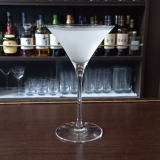(IN\2D%3*2(B 15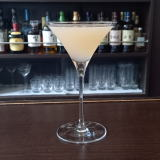(IL+0Y]%4]<^*Y(B 16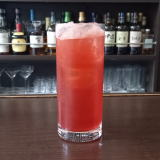(I8W3C^(%=62%X/70(B 17(I2=W%C^%K_I=(B 18\$B5Y\$_(B 19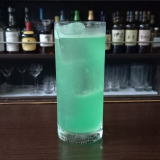(I4RWYD^%80W0(B 20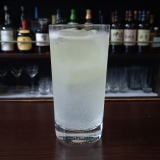(I;O0%@2Q(B 21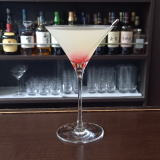(I6<^I(B 22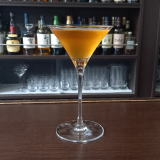(I;]@^0%8W/L_(B 23(ILZ]A(B75 24 \$B5Y\$_(B 25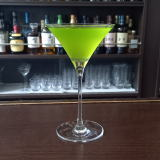(I;O0%L^X0=^(B 26 \$B5Y\$_(B 27(ILWP]:^%ZC^((B 28(IL^W]C^0%8W=@(B 29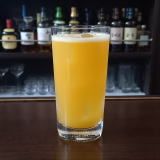(I;O0%L'](B 30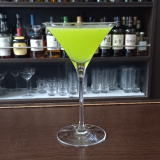(IRSX1Y%8^X0](B 31 \$B5Y\$_(B